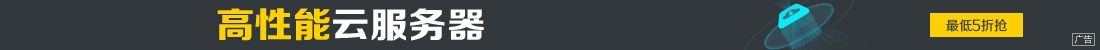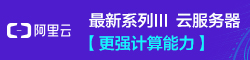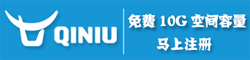# AJax实现类似百度搜索栏的功能 (面试多见)

••```function showHint(str)
{
var xmlhttp;
if (str.length==0)
{
document.getElementByIdx_x("txtHint").innerHTML="";
return;
}
if (window.XMLHttpRequest)
{
xmlhttp=new XMLHttpRequest();
}
else
{
xmlhttp=new ActiveXObject("Microsoft.XMLHTTP");
}
{
{
document.getElementByIdx_x("txtHint").innerHTML=xmlhttp.responseText;
}
}
xmlhttp.open("GET","gethint.asp?q="+str,true);
xmlhttp.send();
}```

```<?php
// 用名字来填充数组
\$a[]="Anna";
\$a[]="Brittany";
\$a[]="Cinderella";
\$a[]="Diana";
\$a[]="Eva";
\$a[]="Fiona";
\$a[]="Gunda";
\$a[]="Hege";
\$a[]="Inga";
\$a[]="Johanna";
\$a[]="Kitty";
\$a[]="Linda";
\$a[]="Nina";
\$a[]="Ophelia";
\$a[]="Petunia";
\$a[]="Amanda";
\$a[]="Raquel";
\$a[]="Cindy";
\$a[]="Doris";
\$a[]="Eve";
\$a[]="Evita";
\$a[]="Sunniva";
\$a[]="Tove";
\$a[]="Unni";
\$a[]="Violet";
\$a[]="Liza";
\$a[]="Elizabeth";
\$a[]="Ellen";
\$a[]="Wenche";
\$a[]="Vicky";
//获得来自 URL 的 q 参数
\$q=\$_GET["q"];
//如果 q 大于 0，则查找数组中的所有提示
if (strlen(\$q) > 0)
{
\$hint="";
for(\$i=0; \$i<count(\$a); \$i++)
{
if (strtolower(\$q)==strtolower(substr(\$a[\$i],0,strlen(\$q))))
{
if (\$hint=="")
{
\$hint=\$a[\$i];
}
else
{
\$hint=\$hint." , ".\$a[\$i];
}
}
}
}
// 如果未找到提示，则把输出设置为 "no suggestion"
// 否则设置为正确的值
if (\$hint == "")
{
\$response="no suggestion";
}
else
{
\$response=\$hint;
}
//输出响应
echo \$response;
?>```